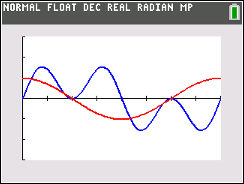# Activities

•• ##### Subject Area

• Math: Geometry: Right Triangles and Trig

9-12

50 Minutes

• ##### Device
•TI-84 Plus CE
•TI-84 Plus CE Python

## Can You Solve a Trig Equation? - IB#### Activity Overview

In this activity, students will practice how to solve equations involving trigonometric functions within a finite interval.

#### Objectives

• Students will solve basic multi-step trigonometric equations within a finite interval.
• Students will review and use trigonometric identities to help in the solving of trigonometric equations.
• Students will try to make a connection with how to understand these topics in IB Mathematics courses and on their final assessments.

#### Vocabulary

• Finite Interval
• Identity
• Verify